### Contest Math II

This quiz is devoted to solving a single problem:

An autobiographical number is a number $N$ such that the first digit of $N$ counts how many zeroes are in $N,$ the second digit counts how many ones are in $N$ and so on. What other autobiographical numbers are there? The autobiographical number 1210 is broken down below as an example.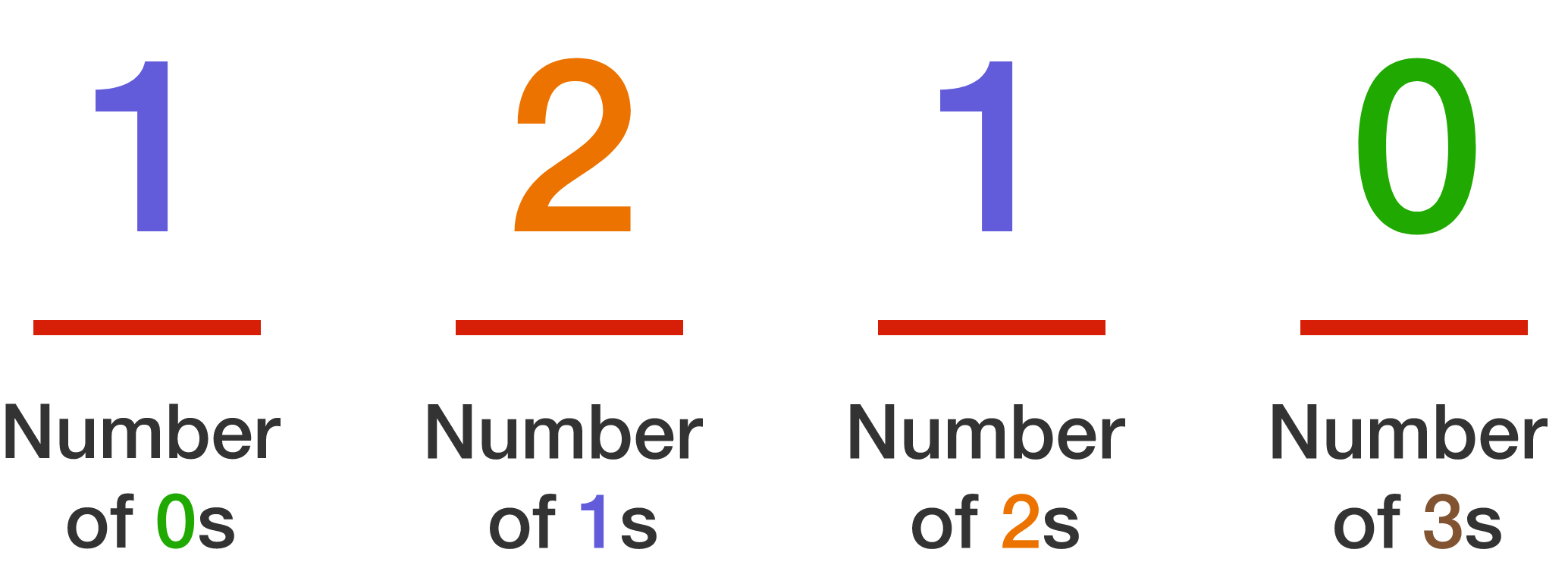We'll start with the 10-digit case, and then go back to the general problem.

Note if you watched the related TED-Ed video (screenshot below) which continues to the solution, this quiz takes a different solving route (and gives hints as to how to extend it) so it will be helpful even if you've seen the rest of the video before.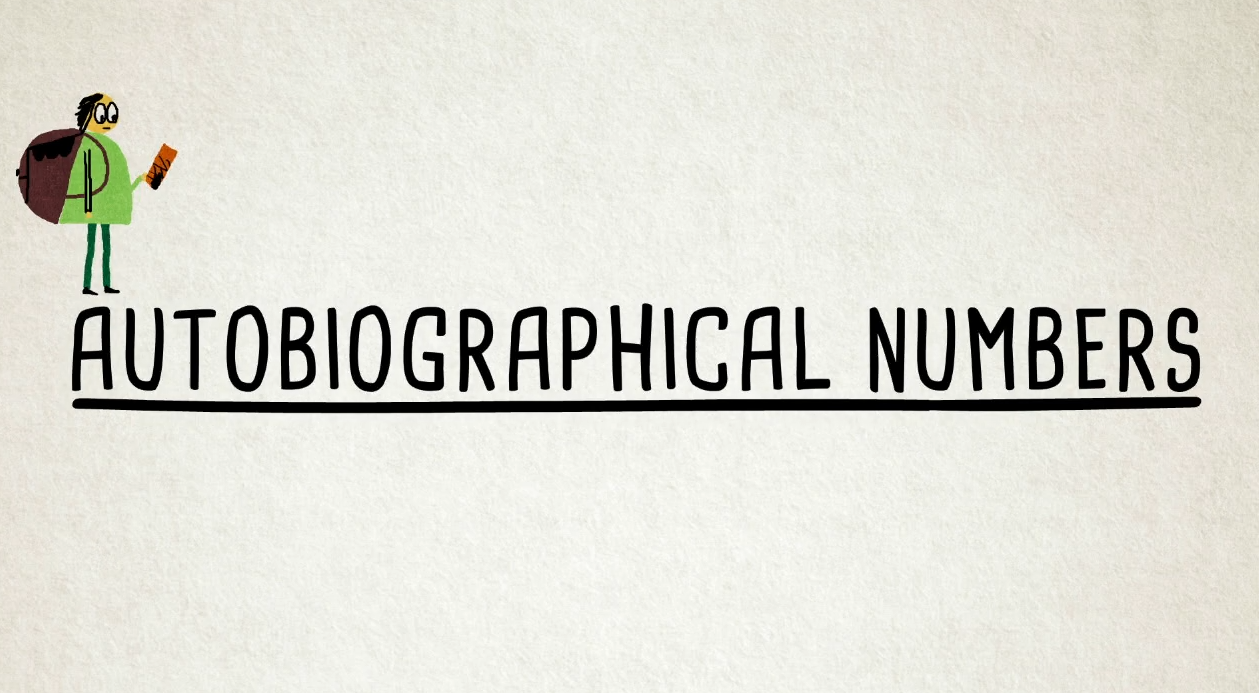# Autobiographical Numbers

An autobiographical number is a number $N$ such that the first digit of $N$ counts how many zeroes are in $N,$ the second digit counts how many ones are in $N$ and so on.

Again, our first goal will be to focus on the question: What is the autobiographical number with 10 digits?

As this problem can be difficult to answer outright, it's broken into parts. First, what is the sum of the 10 digits?

# Autobiographical Numbers

An autobiographical number is a number $N$ such that the first digit of $N$ counts how many zeroes are in $N,$ the second digit counts how many ones are in $N$ and so on.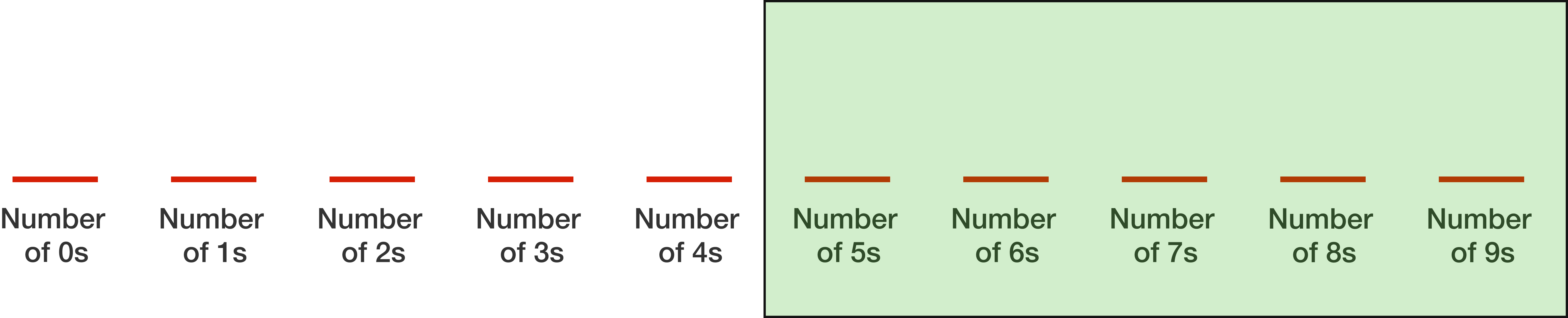Consider the last 5 digits of a 10 digit autobiographical number (as marked above). How many 0 digits must there be in that set?

# Autobiographical Numbers

An autobiographical number is a number $N$ such that the first digit of $N$ counts how many zeroes are in $N,$ the second digit counts how many ones are in $N$ and so on.

Using the previous answer, we know the latter digits must be all 0s (except for a 1 somewhere; the extra digit can't be more than 1 because that will cause the overall sum of digits to exceed the target of 10).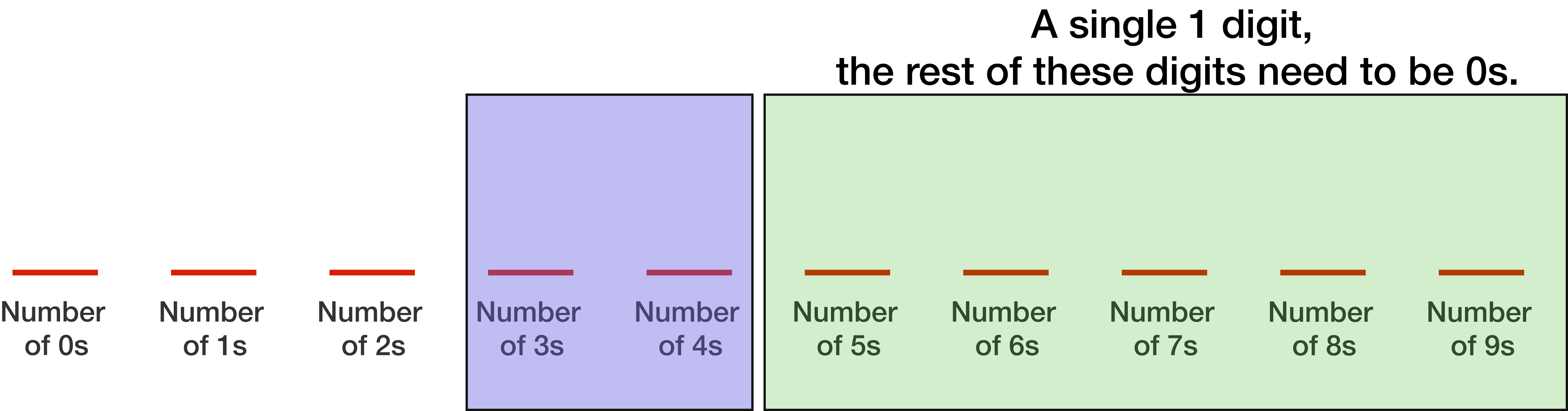What about the 3s and 4s digit places (as marked above)?

# Autobiographical Numbers

To summarize the previous results (including information from the solutions), we know the sum of the digits must be 10, and the facts from this diagram: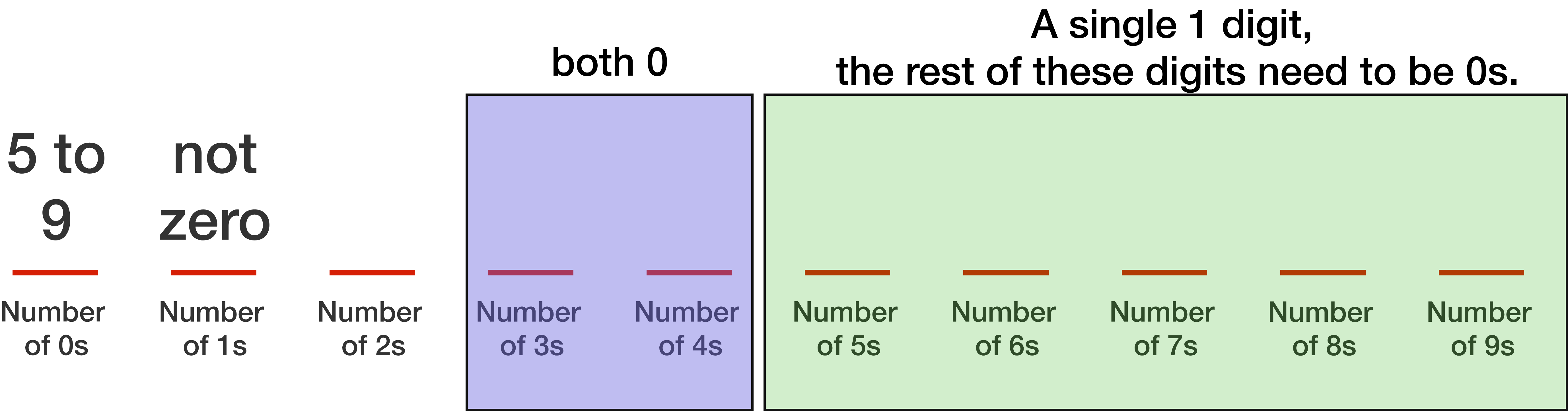We're close to an answer now!

# Autobiographical Numbers

An autobiographical number is a number $N$ such that the first digit of $N$ counts how many zeroes are in $N,$ the second digit counts how many ones are in $N$ and so on.What is the first digit of the 10 digit autobiographical number? That is, how many digits of the digits in an 10 digit autobiographical number must be 0?

# Autobiographical Numbers

An autobiographical number is a number $N$ such that the first digit of $N$ counts how many zeroes are in $N,$ the second digit counts how many ones are in $N$ and so on.Combine the information from the previous questions, and you should now be able to answer:

What is the autobiographical number with 10 digits?

# Autobiographical Numbers

Now, how to solve the general case?

Our first steps can apply in a general way. For example, when checking 8-digit autobiographical numbers, this diagram arises.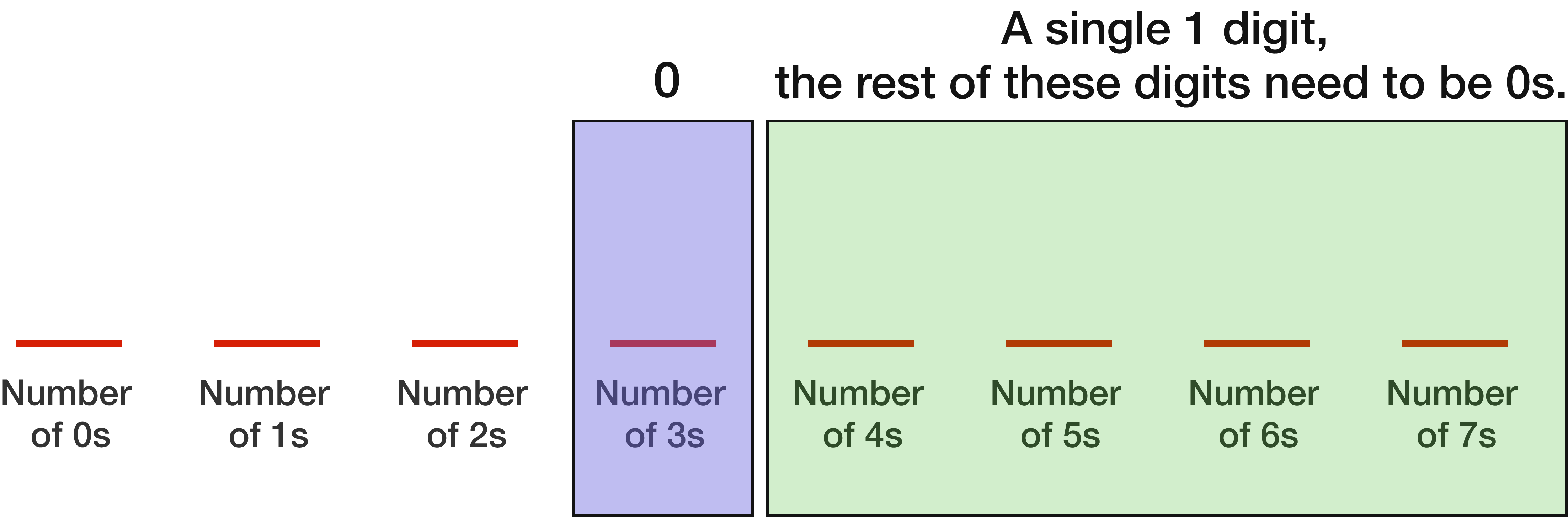From these steps it's possible to get a general formula for the first digit for when $4 \leq N \leq 10 .$

Once you have this information, you have enough constraints that the different cases fall very quickly. Note, as a word of warning:

• At least one of the cases of $N$ has two numbers that fit rather than one.

• At least one of the cases of $N$ has no numbers of that length.

×